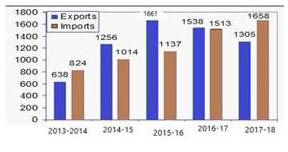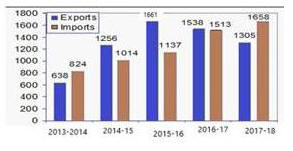# 04 July 2019 – Evening-Shift (Quantitative Aptitude)

SSC CHSL (Tier – 1) Online Exam Paper – 2018 “held on 04 JULY 2019”

Evening-Shift (Quantitative Aptitude)

Q1. 9 years ago, the average age of a family of five members was 33 years. Now, three new members join whose ages are in ascending order with consecutive gaps of 8 years. If the present average age of the family is the same as it was 9 years ago, what is the age (in years) of the youngest new member?

Options:

1. 17
2. 9
3. 15
4. 10

Q2. There was 29% off on bags. A lady bought a bag and got 13% discount for paying in cash. She paid Rs.671.70. What was the price tag (in Rs) on the bag?

Options:

1. 750
2. 800
3. 1000
4. 925

Q3. A train covers 60 km at a uniform speed. If the speed had been 8 km/h more, it would have taken 10 hours less for the same journey. What is the speed of the train (in km/h) ?

Options:

1. 4
2. 5
3. 3
4. 2.5

Q4. The given Bar Graph presents the Imports and Exports of an item (in tonnes) manufactured by a company for the five financial years, 2013-2014 to 2017-2018.What is the ratio of total Exports to total Imports during the five financial years ?

Options:

1. 4175 : 4011
2. 4011 : 4175
3. 3073 : 3199
4. 3199 : 3073

Correct answer : 3199 : 3073

Q5. A,B and C can complete a piece of work in 4, 28 and 56 days respectively. working together, they can complete the same work in how many days?

Options:

1. 90/17
2. 56/17
3. 86/17
4. 52/17

Q6. In triangle ABC, D and E are two points on the sides AB and AC respectively so that DE || BC and AD/BD = 3/4. The ratio of the area of ∠ABC to the area of trapezium DECB is :

Options:

1. 40 : 49
2. 49 : 40
3. 49 : 33
4. 33 : 49

Correct answer : 49 : 40

Q7. If (3x – 7)³ + (3x – 8)³ + (3x + 6)³ =
3 (3x – 7) + (3x – 8) + (3x + 6), then what is the value of x?

Options:

1. 3
2. 2
3. 4
4. 1

Q8. A circle is inscribed in a triangle ABC. It touches sides AB, BC and AC at the points P, Q and R respectively. If BP = 8.5 cm, CQ = 6.5 cm and AR = 4.5 cm, then the perimeter (in cm) of the ∠ABC is:

Options:

1. 49.5
2. 39
3. 33
4. 35

Q9.  If a² + b² =99 and ab -11, (a > 0, b > 0) then the value of (a³ + b³) is:

Options:

1. 968
2. 1250
3. 1080
4. 1100

Q10. The given Bar Graph presents the Imports and Exports of an item (in tonnes) manufactured by a company for the five financial years, 2013-2014 to 2017-2018.In which financial year the absolute difference between the Exports and the Imports is the highest ?

Options:

1. 2015 – 2016
2. 2016 – 2017
3. 2017 – 2018
4. 2014 – 2015

Correct answer : 2015 – 2016

Q11. Two circles of radii 7 cm and 9 cm intersect at the points A and B. If AB = 6 cm, and the distance between the centres of the circles is x cm, then the value of x (to the closest integer) is:

Options:

1. 10
2. 15
3. 12
4. 14

Q12. If  x4 + X¯4 = 1442, (x>0) then the value of x + x¯ is:

Options:

1. 15
2. 6
3. 8
4. 7

Q13. A man gets a discount of 30% and then 20% on his food bill of Rs.1,500. How much discount, in rupees, did he get ?

Options:

1. 500
2. 660
3. 360
4. 700

Q14. If x + 1/x = 10 , then x³ + 1/x³ is equal to:

Options:

1. 1030
2. 1000
3. 1100
4. 970

Q15. If 2 sinθ² + 5 cosθ – 4 = 0,0° < θ < 90°, then the value of tanθ + sinθ is:

Options:

1. 2√3
2. 3√3/2
3. √3/3
4. √3/2

Q16. A certain amount invested at a certain rate, compounded annually, grows to an amount in five years, which is a factor of 1.1881 more than to what it would have grown in three years. What is the rate percentage?

Options:

1. 8.1
2. 8
3. 9
4. 9.2

Q17. A man bought 2 articles for Rs.3050 each. He sold article at 10% profit and another at 20% profit. The total profit percentage he earned is:

Options:

1. 18%
2. 15%
3. 20%
4. 10%

Q18. The given Bar Graph presents the Imports and Exports of an item (in tonnes) manufactured by a company for the five financial years, 2013-2014 to 2017-2018.In which financial year the percentage increase in Imports and Exports taken together is the highest is the highest in comparison to its previous financial year?

Options:

1. 2014 – 2015
2. 2017 – 2018
3. 2015 – 2016
4. 2016 – 2017

Correct answer : 2014 – 2015

Q19.  Three numbers are in the ratio 1/2 : 2/3 : 3/4. The difference between the greatest and the smallest number is 27. The smallest number is:

Options:

1. 72
2. 54
3. 81
4. 40

Q20.  Let ∠ABC ~ ∠QPR and ar(∠ABC)/ar(∠QPR) = 1/16. If AB = 12cm, BC = 6cm and AC = 9 cm then PR is equal to:

Options:

1. 24cm
2. 9cm
3. 8cm
4. 12cm

Q21. What is the area of a rhombus (in cm²) whose side is 13 cm and the smaller diagonal is 10 cm ?

Options:

1. 96
2. 120
3. 192
4. 50

Q22. If cosθ = 2p / p²+1,(p ≠ 0)then sin is equal to:

Options:

1. 2p / p²-1
2. p²+1/ p²-1
3. 2p / p² +1
4. p²-1/2p

Q23. A number is increased by 30%, then decreased by 30%, and the further increased by 10%. what is the net increase/decrease percent in the number (correct to the nearest integer)

Options:

1. 19% increase
2. 18% decrease
3. 19% decrease
4. 18% increase

Q24. In a ∠ABC, the bisectors of ∠B and ∠C meet at point O within the triangle. If ∠BOC is given, then which among the given options is true?

Options:

1.∠A=180° + ∠BOC
2.∠A=90° + ∠BOC
3.∠A= 2(90°- ∠BOC)
4.∠A= 2(∠BOC – 90°)

Correct answer : ∠A= 2(∠BOC – 90°)

Q25. The given Bar Graph presents the Imports and Exports of an item (in tonnes) manufactured by a company for the five financial years, 2013-2014 to 2017-2018.What is the average of Import () during the five financial years?

Options:

1. 1335.9
2. 1229.5
3. 1229.2
4. 1552.4